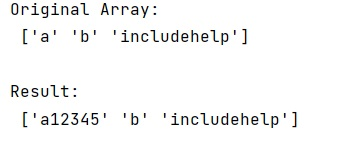# How to create a numpy array of arbitrary length strings?

Learn, how to create a numpy array of arbitrary length strings in Python?
Submitted by Pranit Sharma, on January 09, 2023

NumPy is an abbreviated form of Numerical Python. It is used for different types of scientific operations in python. Numpy is a vast library in python which is used for almost every kind of scientific or mathematical operation. It is itself an array which is a collection of various methods and functions for processing the arrays.

## Creating a numpy array of arbitrary length strings

A string is a group of characters, these characters may consist of all the lower case, upper case, and special characters present on the keyboard of a computer system. A string is a data type and the number of characters in a string is known as the length of the string.

For this purpose, we will create an array of dtype=object. If we try to assign a long string to a normal NumPy array, it truncates the string.

Let us understand with the help of an example,

## Python code to create a numpy array of arbitrary length strings

```# Import numpy
import numpy as np

# Creating an array
arr = np.array(['a', 'b', 'includehelp'], dtype='object')

# Display original array
print("Original Array:\n",arr,"\n")

# to numpy array elements
arr += '12345'

# Display result
print("Result:\n",arr,"\n")
```

Output: